# Civil Engineering - GATE Exam Questions

### Exercise :: GATE Exam Questions - Section 1

36.

The depth of tension crack in a soft clay (φu = 0) is

 A.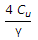B.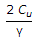C.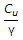D.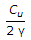Explanation:

No answer description available for this question. Let us discuss.

37.

A single rapid test to determine the pollu-tional status of river water is :

 A. biochemical oxygen demand B. chemical oxygen demand C. total organic solids D. dissolved oxygen

Explanation:

No answer description available for this question. Let us discuss.

38.

The vertical stress at depth z, directly below the point load p is (k is a constant)

 A.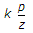B.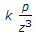C.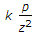D.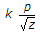Explanation:

No answer description available for this question. Let us discuss.

39.

Vane tester is normally used for determining in situ shear strength of :

 A. soft clays B. sand C. stiff clays D. gravel

Explanation:

No answer description available for this question. Let us discuss.

40.

For the frame shown in the below figure, the maximum bending moment in the column is,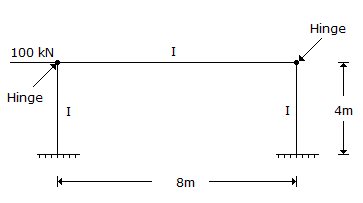A. zero B. 400 kNm C. 100 kNm D. 200 kNm